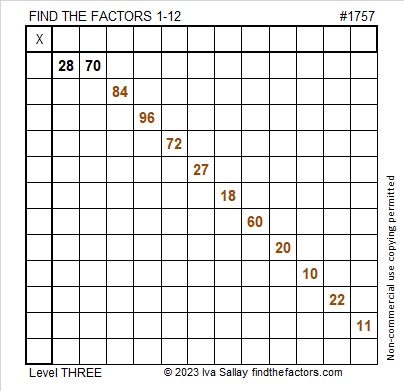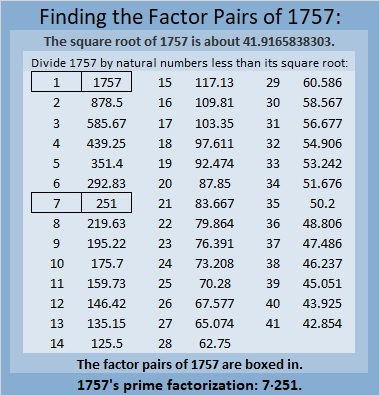# 1757 A Shillelagh Puzzle

Contents

### Today’s Puzzle:

When I was in elementary school, one of my teachers had us listen to a variety of songs for Saint Patrick’s Day including Bing Crosby’s It’s the Same Old Shillelagh. It’s almost Saint Patrick’s Day, so here’s a puzzle that looks a little like a shillelagh, an Irish walking stick.

Since this is a level-3 puzzle, begin with the clues at the top of the puzzle and work your way down using each number 1 to 12 once in the first column and once in the top row. The numbers you write and the given clues need to form a multiplication table. There is only one solution.Here is the same puzzle in black and white:### Factors of 1757:

• 1757 is a composite number.
• Prime factorization: 1757 = 7 × 251.
• 1757 has no exponents greater than 1 in its prime factorization, so √1757 cannot be simplified.
• The exponents in the prime factorization are 1 and 1. Adding one to each exponent and multiplying we get (1 + 1)(1 + 1) = 2 × 2 = 4. Therefore 1757 has exactly 4 factors.
• The factors of 1757 are outlined with their factor pair partners in the graphic below.### More About the Number 1757:

1757 is the difference of two squares in two different ways:
879² – 878² = 1757, and
129² – 122² = 1757.

1757 is palindrome 616 in base17
because 6(17²) + 1(17) + 6(1) = 1757.

This site uses Akismet to reduce spam. Learn how your comment data is processed.